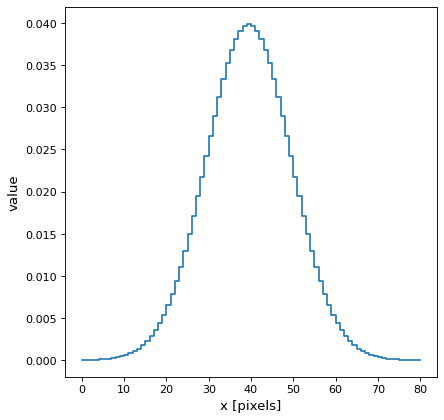# Gaussian1DKernel¶

class `astropy.convolution.``Gaussian1DKernel`(stddev, **kwargs)[source]

1D Gaussian filter kernel.

The Gaussian filter is a filter with great smoothing properties. It is isotropic and does not produce artifacts.

The generated kernel is normalized so that it integrates to 1.

Parameters
stddevnumber

Standard deviation of the Gaussian kernel.

x_size`int`, optional

Size of the kernel array. Default = ⌊8*stddev+1⌋.

mode`str`, optional
One of the following discretization modes:
• ‘center’ (default)

Discretize model by taking the value at the center of the bin.

• ‘linear_interp’

Discretize model by linearly interpolating between the values at the corners of the bin.

• ‘oversample’

Discretize model by taking the average on an oversampled grid.

• ‘integrate’

Discretize model by integrating the model over the bin. Very slow.

factornumber, optional

Factor of oversampling. Default factor = 10. If the factor is too large, evaluation can be very slow.

Examples

Kernel response:

```import matplotlib.pyplot as plt
from astropy.convolution import Gaussian1DKernel
gauss_1D_kernel = Gaussian1DKernel(10)
plt.plot(gauss_1D_kernel, drawstyle='steps')
plt.xlabel('x [pixels]')
plt.ylabel('value')
plt.show()
```

(png, svg, pdf)